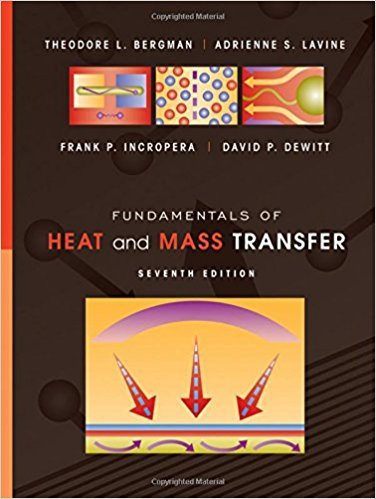×

# The one-dimensional plane wall of Figure 3.1 isISBN: 9780470501979 82

## Solution for problem 9.1 Chapter 9

Fundamentals of Heat and Mass Transfer | 7th Edition

• Textbook Solutions
• 2901 Step-by-step solutions solved by professors and subject experts
• Get 24/7 help from StudySoup virtual teaching assistantsFundamentals of Heat and Mass Transfer | 7th Edition

4 5 0 280 Reviews
31
3
Problem 9.1

The one-dimensional plane wall of Figure 3.1 is ofthickness L?75 mm and thermal conductivity k?5W/m?K. The fluid temperatures are T?,1?200?C and T?,2?100?C, respectively. Using the minimum andmaximum typical values of the convection heat trans-fer coefficients listed in Table 1.1, determine the mini-mum and maximum steady-state heat fluxes throughthe wall for (i) free convection in gases, (ii) free con-vection in liquids, (iii) forced convection in gases, (iv)forced convection in liquids, and (v) convection withphase change

Step-by-Step Solution:
Step 1 of 3
Step 2 of 3

Step 3 of 3

##### ISBN: 9780470501979

The full step-by-step solution to problem: 9.1 from chapter: 9 was answered by , our top Engineering and Tech solution expert on 09/04/17, 10:17PM. This full solution covers the following key subjects: Convection, heat, liquids, Wall, gases. This expansive textbook survival guide covers 14 chapters, and 1569 solutions. This textbook survival guide was created for the textbook: Fundamentals of Heat and Mass Transfer, edition: 7. Fundamentals of Heat and Mass Transfer was written by and is associated to the ISBN: 9780470501979. The answer to “The one-dimensional plane wall of Figure 3.1 is ofthickness L?75 mm and thermal conductivity k?5W/m?K. The fluid temperatures are T?,1?200?C and T?,2?100?C, respectively. Using the minimum andmaximum typical values of the convection heat trans-fer coefficients listed in Table 1.1, determine the mini-mum and maximum steady-state heat fluxes throughthe wall for (i) free convection in gases, (ii) free con-vection in liquids, (iii) forced convection in gases, (iv)forced convection in liquids, and (v) convection withphase change” is broken down into a number of easy to follow steps, and 74 words. Since the solution to 9.1 from 9 chapter was answered, more than 250 students have viewed the full step-by-step answer.

Unlock Textbook Solution

The one-dimensional plane wall of Figure 3.1 is

×